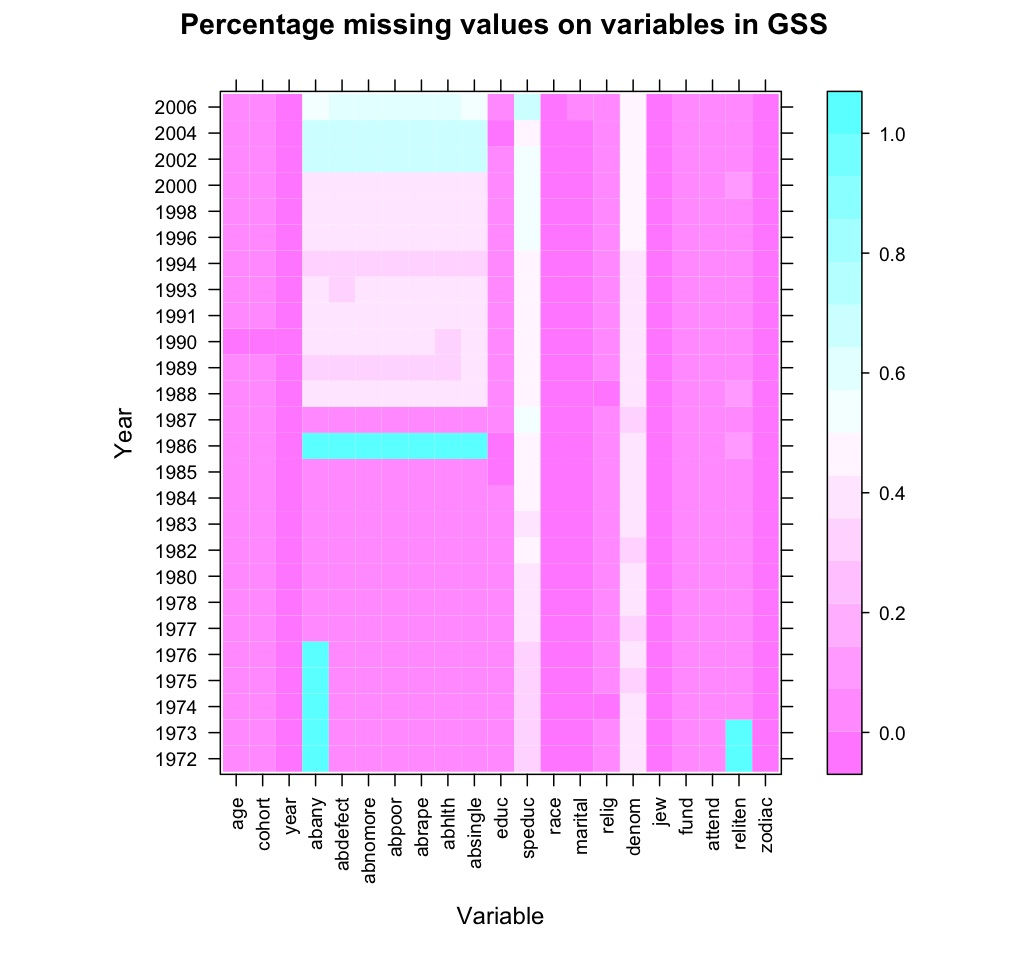# R-Sessions 30: Visualizing missing values

It always takes some time to get a grip on a new dataset, especially large ones. The code-books are often as indispensable as they are massive, and not always as clear as one would want. Routings, and resulting and strange patterns of missing values are at times difficult to find.

I found a nice way to plot missing values, using R. Basically, I thought it would be nice to calculate the percentage of missings on each variable, and do so for each year represented in the data. These numbers could be visualized using a levelplot(), which resulted in the graph below.In this example I used a small subset of variables from the cumulative file of the General Social Survey, which is freely available from the web. I used this syntax:

``` testing.NA <- matrix(ncol=26, nrow=21) for (i in 1:dim(GSS)) { testing.NA[i,] <- tapply(GSS[[i]], GSS\$year, function(x) sum(is.na(x)) / length(x)) }```

``` dimnames(testing.NA) <- list( names(GSS), sort(unique(GSS\$year))) library(lattice) ```

```levelplot(testing.NA, scales=list(x=list(rot=90)), main="Percentage missing values on variables in GSS", xlab="Variable", ylab="Year") ```

First, I defined the testing.NA matrix, using the number of years and variables. Then, in a loop, I calculate the percentage missing values, basically using is.na() and length(). I assign dimnames to the matrix and use the levelplot() function from the lattice-library to plot the matrix. That's it, easy does it.

But: does it help? I think it does. Of course, all this information can be gained from the code-book, and needs to be verified. However, it does give us some immediate notes on the availability of these variables. For instance, we see that in the first few years, the abany variable is missing, whereas other variables on abortion don't. When creating scales this needs to be taken into account, not to lose the complete data on the first few years. The speduc-variable (spouse's educational level) has a high number of missings, as does the denom variable. This, however, makes sense: not everybody has a spouse and the denom-variable only applies to protestants. Finally, this graph gives some pointers on a change in survey-strategy from 1988 onwards regarding the items on induced abortion. The percentage missing values increased sharply at that point, and does so for all abortion-related variables.

This graph does not tell what exactly happened, but does provides nice pointers on what to look for when reading the code-book.